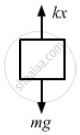# A Block of 2 Kg is Suspended from a Ceiling by a Massless Spring of Spring Constant K = 100 N/M. What is the Elongation of the Spring? If Another - Physics

Sum

A block of 2 kg is suspended from a ceiling by a massless spring of spring constant k = 100 N/m. What is the elongation of the spring? If another 1 kg is added to the block, what would be the further elongation?

#### Solution

Given,
mass of the first block, m = 2 kg
k = 100 N/m
Let elongation in the spring be x.From the free-body diagram,
kx = mg
$x = \frac{mg}{k} = \frac{2 \times 9 . 8}{100}$
$= \frac{19 . 6}{100} = 0 . 196 \approx 0 . 2 m$
Suppose, further elongation, when the 1 kg block is added, is $∆ x$ Then, $k\left( x + ∆ x \right) = m'g$
⇒ k  $∆ x$ 3g − 2g = g
$\Rightarrow ∆ x = \frac{g}{k} = \frac{9 . 8}{100} = 0 . 098 \approx 0 . 1 m$

Concept: Newton's First Law of Motion
Is there an error in this question or solution?

#### APPEARS IN

HC Verma Class 11, Class 12 Concepts of Physics Vol. 1
Chapter 5 Newton's Laws of Motion
Q 17 | Page 80